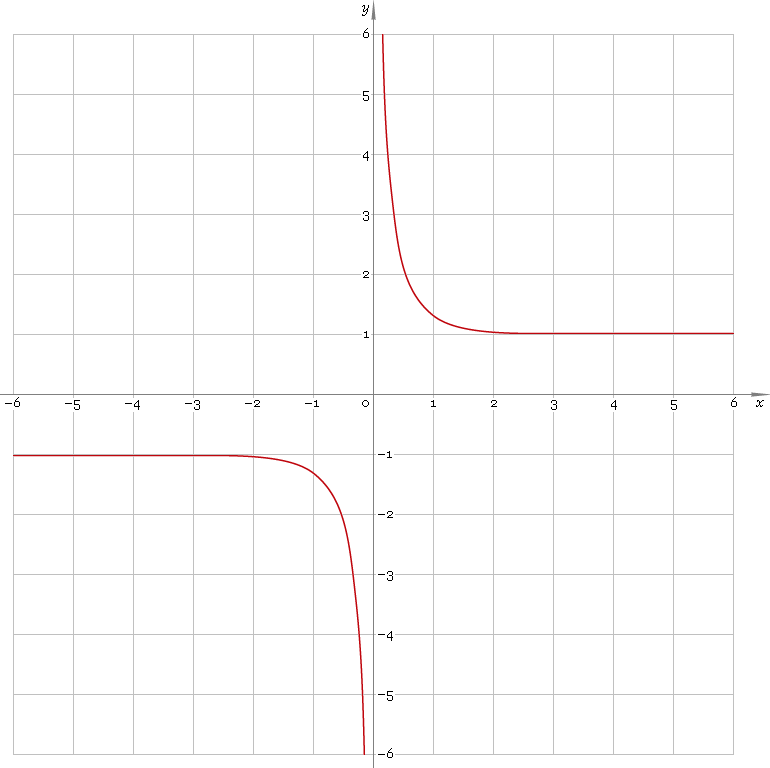The Art of Mathematics

# coth or cth — hyperbolic cotangent function

## 1. Definition

Hyperbolic cotangent is defined as

cothx ≡ (ex + ex) /(ex − ex)

## 2. Graph

Hyperbolic cotangent is antisymmetric function defined everywhere on real axis, except its singular point 0 — so function domain is (−∞, 0)∪(0, +∞). Its graph is depicted below — fig. 1.Fig. 1. Graph of the hyperbolic cotangent function y = cothx.

Function codomain is entire real axis with a gap in the middle: (−∞, −1)∪(1, +∞).

## 3. Identities

Base:

coth2x − csch2x = 1

By definition:

cothx ≡ coshx /sinhx ≡ 1 /tanhx

Property of antisymmetry:

coth−x = −cothx

Half-argument:

coth(x/2) = (1 + coshx) /sinhx
coth(x/2) = sinhx /(coshx − 1)
cothx = [1 + tanh2(x/2)] /[2 tanh(x/2)]

Doulbe argument:

coth(2x) = (coth2x + 1) /(2 cothx)

Triple argument:

coth(3x) = (coth3x + 3 cothx) /(3 coth2x + 1)

coth(4x) = (coth4x + 6 coth2x + 1) /(4 coth3x + 4 cothx + 1)

Power reduction:

coth2x = (cosh(2x) + 1) /(cosh(2x) − 1)
coth3x = (cosh(3x) + 3 coshx) /(sinh(3x) − 3 sinhx)
coth4x = (cosh(4x) + 4 cosh(2x) + 3) /(cosh(4x) − 4 cosh(2x) + 3)
coth5x = (cosh(5x) + 5 cosh(3x) + 10 coshx) /(sinh(5x) − 5 sinh(3x) + 10 sinhx)

Sum and difference of arguments:

coth(x + y) = (1 + cothx cothy) /(cothx + cothy)
coth(xy) = (1 − cothx cothy) /(cothx − cothy)

Product:

cothx cothy = [cosh(x + y) + cosh(xy)] /[cosh(x + y) − cosh(xy)]

Sum:

cothx + cothy = sinh(x + y) /(sinhx sinhy)
cothx − tanhy = sinh(yx) /(sinhx sinhy)

## 4. Derivative and indefinite integral

Hyperbolic cotangent derivative:

coth′x = −csch2x ≡ −1 /sinh2x

Indefinite integral of the hyperbolic cotangent:

∫ cothx dx = ln|sinhx| + C

where C is an arbitrary constant.

## 5. How to use

To calculate hyperbolic cotangent of the number:

``coth(−1);``

To get hyperbolic cotangent of the complex number:

``coth(−1+i);``

To get hyperbolic cotangent of the current result:

``coth(rslt);``

To get hyperbolic cotangent of the number z in calculator memory:

``coth(mem[z]);``

## 6. Support

Hyperbolic cotangent of the real argument is supported in free version of the Librow calculator.

Hyperbolic cotangent of the complex argument is supported in professional version of the Librow calculator.